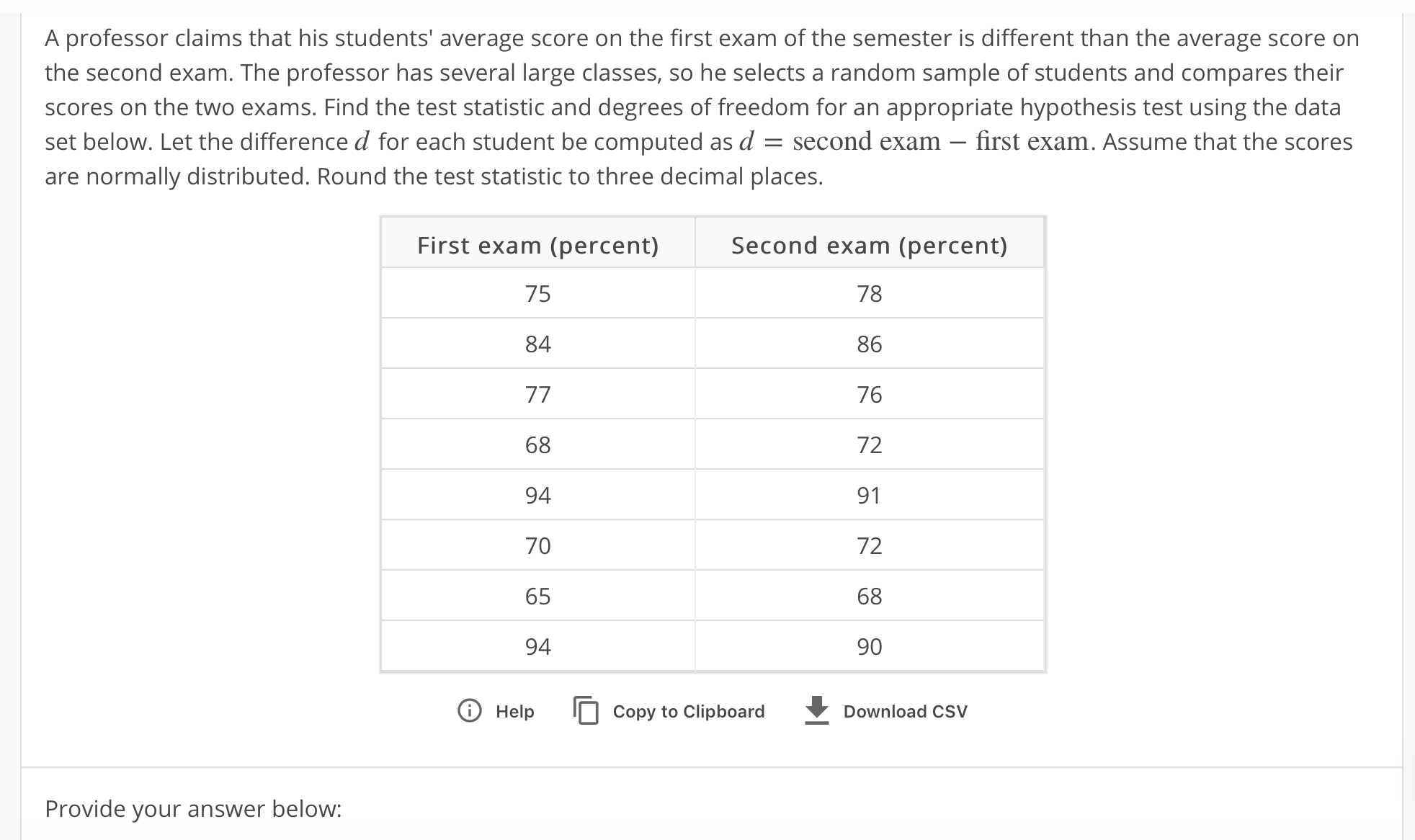A professor claims that his students' average score on the first exam of the semester is different than the average score ornthe second exam. The professor has several large classes, so he selects a random sample of students and compares theirscores on the two exams. Find the test statistic and degrees of freedom for an appropriate hypothesis test using the dataset below. Let the difference for each student be computed as đ second exam-first exam. Assume that the scoresare normally distributed. Round the test statistic to three decimal places.Second exam (percent)7886767291726890First exam (percent)75846894706594T Help Copy to ClipboardDownload CSVProvide your answer below

Question

T=

DF=help_outlineImage TranscriptioncloseA professor claims that his students' average score on the first exam of the semester is different than the average score orn the second exam. The professor has several large classes, so he selects a random sample of students and compares their scores on the two exams. Find the test statistic and degrees of freedom for an appropriate hypothesis test using the data set below. Let the difference for each student be computed as đ second exam-first exam. Assume that the scores are normally distributed. Round the test statistic to three decimal places. Second exam (percent) 78 86 76 72 91 72 68 90 First exam (percent) 75 84 68 94 70 65 94 T Help Copy to Clipboard Download CSV Provide your answer below fullscreen
Step 1

Paired t-test statistic:

In order to test a hypothesis regarding whether the difference between a paired set of n observations (xi, yi) is significant or not, the paired t-test is used. The test statistic for the paired t-test is given as follows:

Step 2

Calculation:

The following table shows the necessary calculations for finding the test statistic:

Step 3

Further calculations:...

Want to see the full answer?

See Solution

Want to see this answer and more?

Our solutions are written by experts, many with advanced degrees, and available 24/7

See Solution
Tagged in

Hypothesis Testing